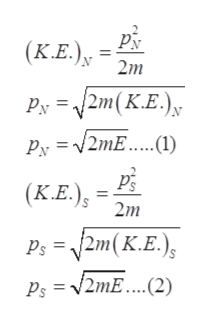# A car has the same kinetic energy when travelling north as when it turns around and travels south. Is the momentum of car same in both cases?

Question
40 views

A car has the same kinetic energy when travelling north as when it turns around and travels south. Is the momentum of car same in both cases?

check_circle

Step 1

Let, Kinetic energy = E

Kinetic energy in north = kinetic energy in south = E

Step 2

Relation between the kinetic energy and the momentum can be given ashelp_outlineImage TranscriptionclosePN (K.E.), 2m 2m(K.E.) Py = Py = V2ME.(1) (K.E.), 2m(K.E.); Ps = Ps = V2ME.…...(2) fullscreen
Step 3

From equation (1...

### Want to see the full answer?

See Solution

#### Want to see this answer and more?

Solutions are written by subject experts who are available 24/7. Questions are typically answered within 1 hour.*

See Solution
*Response times may vary by subject and question.
Tagged in

### Work,Power and Energy